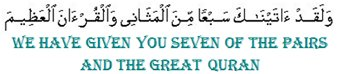15:87

The significance of 15:87 was kept hidden for 14 centuries. Up until recently, the traditional interpretation of this verse was that it refers to the Fatiha and its seven verses, being described as the most repeated verses of the Quran.
To justify this interpretation, the word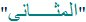(al-mathani) in 15:87 was translated as "oft-repeated".

The following is one example of the traditional translations of 15:87:

We have given thee seven of the oft-repeated (verses) and the Great Qu'ran
(M.M.Pickthall)

Apart from 15:87, the word 'mathani" (plural) is also used in 39:23. As for the singular 'mathna', it is used in three verses: 4:3, 34:46 and 35:1.
When we study these verses we find that in every one of them, the words 'mathani' (plural) and 'mathna' (singular) mean pairs and pair respectively. None of these verses support the 'oft-repeated' interpretation.

To justify the interpretation that refers to the 7 verses of the Fatiha, we find that the majority of the traditional translations added the word 'verses' between brackets. The word 'verses' is not found in the Arabic text.

Since the word 'mathani' is always used in the Quran to mean pairs, the words in 15:87 speak about 7 pairs. The question is what are the 7 pairs?

The discovery of the correct meaning of 15:87 was made by Rashad Khalifa. Now we know that the seven pairs (14) relate to the 14 letters of the Arabic alphabet that are used in different combinations at the beginning of 29 Suras in the Quran.

Now we also know that the 14 letters constitute an integral feature of the mathematical design of the Quran based on the number 19.
For more details about the role of the 14 letters within the mathematical design of the Quran, please go to:
Miracle of the Quran

----------------------------------------
The research below shows how the words in 15:87 provide an intricate design in themselves.
Ultimately, the design presented below confirms that the words: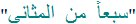(seven of the pairs) in 15:87 have nothing to do with the 7 verses of the Fatiha, but indeed relate to the 14 letters which are one of the many features of the miracle of 19 in the Holy Quran.

1-
Sura 15, which starts with abbreviated letters, has 99 verses. This is the only Sura in the Quran in which the sum of the Sura number and number of verses add up to the number of Suras in the Quran.
15 + 99 = 114 (number of suras in the Quran).

In verse 1, we have three abbreviated letters. These are followed with the words:
These are the signs of the Book
There are 86 verses between verse 1 (which confirms that the abbreviated letters are the signs/miracles of the Book) and verse 87 where we have specific mention of the 14 letters (7 pairs).
8 + 6 = 14

2-
The Gematrical Value (GV) of the word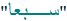(seven) is 133 (19 x 7)
Note that the number (seven) is identical to the co-efficient (7).
If we add the digits of 133 we also get 7:
1 + 3 + 3 = 7
The two sevens (co-efficient and the sum of digits) add up to 14 (7 pairs)
p.s. A table showing the Gematrical Values (GV) of the Arabic letters is found at the bottom of this article.

3-
The GV of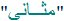(pairs) is 601
6 + 0 + 1 = 7

4- The GV of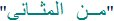(of the pairs) is 722
If we multiply the digits of 722:
7 x 2 x 2 = 28
28 = 7 Pairs x 2
Those correspond to the 14 abbreviated letters + the 14 combinations of letters that are used in the beginning of some Suras

5-
The GV of(of the pairs) as we have seen is 722
722 = 19 x 19 x 2
The double 19 (pair) and the remaining 2 (pair) are strong reminders that these words (of the pairs) relate to the code 19 in the Quran and not the oft-repeated verses.

6- The following table shows the GV of the 14 letters:

--------------Table 1
 Letter GV A (Alef) 1 L (Laam) 30 M (Meem) 40 S (Saad) 90 R (Ra) 200 K (Kaf) 20 H (Ha) 5 Y (Ya) 10 Aa (Ayn) 70 Tt (Ta) 9 S (Seen) 60 H (Ha) 8 Q (Qaf) 100 N (Noon) 50 Total 693

The total GV of the 14 letters (7 pairs) is 693

If we add the two numbers, (693) and (29) we get:
693 + 29 = 722
The number 722 is of course the GV of the words(of the pairs) in 15:87. This is a definite link that connects the 7 pairs mentioned in 15:87 to the 14 letters.

7- The 14 letters of the alphabet are used in different combinations at the beginning of 29 Suras, such as A.L.M and A.L.R and others. The number of different combinations is also 14.
And so, we have 14 letters, 14 combinations, 29 Suras.
The total of the 3 numbers (14+14+29) = 57

The GV of(seven) = 133
The GV of(of the pairs) = 722
Both of the above numbers, 133 and 722, are multiples of 19
133 = 19 x 7
722 = 19 x 19 x 2
Let us look at these coefficients, (19 / 7) and also (19 / 19 / 2)
a- If we add the three 19's we get 57
As we have seen above, 57 corresponds to the 14 letters + 14 combinations + 29 Suras

8-
Now let us multiply the three 19's, we get 6859
6 + 8 + 5 + 9 = 28
28 = 14 letters + 14 combinations
also 6 + 8 = 14
and 5 + 9 = 14
And if we multiply the remaining coefficients, 7 and 2:
7 x 2 = 14 (7 pairs)

9- To recall, the GV of(seven) = 133
And the GV of(of the pairs) = 722
Let us now multiply the two GV:
133 x 722 = 96026
The number 96026 possesses a peculiar property that is also connected to the 7 pairs (14). Every time we divide 96026 by 19 we get numbers that reduce to 14
96026 divided by 19 = 5054
5 + 0 + 5 + 4 = 14 (7 pairs)
If we divide the resulting number (5054) by 19 again:
5054 divided by 19 = 266
2 + 6 + 6 = 14 (7 pairs)
If we divide the resulting number (266) by 19 again, we get 14

------------------------------------------

The parameters of(seven of the pairs) are represented in the following table:

-------------------------Table 2
 Words 3 Letters 13 GV of 3 words 855 Unrepeated letters 9 GV of unrepeated letters 763

The patterns to follow are based on the data in table 2

10-
Number of words + unrepeated letters + GV of unrepeated letters
3 + 9 + 763 = 775
7 + 7 + 5 = 19

11- By adding the individual digits above (3) (9) (763) we get:
3 + 9 + 7 + 6 + 3 = 28
28 = 14 (7 pairs) x 2
These correspond to the 14 letters and 14 combinations

12- Number of words + number of letters + GV of unrepeated letters
3 + 13 + 763 = 779
= 19 x 41

13-
If we add all five parameters:
3 + 13 + 855 + 9 + 763 = 1643
1 + 6 + 4 + 3 = 14 (7 pairs)

14- The GV of the word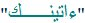(We have given you) is 482
4 + 8 + 2 = 14 (7 pairs)

15- The GV of the 5 words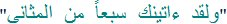(We have given you seven of the pairs) is 1477
1 + 4 + 7 + 7 = 19

16- The GV of the 5 words + the number of words (5)
1477 + 5 = 1482
= 19 x 78
Since the true meaning of 15:87 was decreed by God to be unveiled by Rashad Khalifa, we find that numerical code of Rashad Khalifa (GV of his name), which is 1230, also woven into the pattern of 15:87

Observation
While as the majority of the facts above yielded the number 14, so as to confirm that the words in 15:87 speak of 7 pairs and not oft-repeated verses, the calculations to follow, which incorporates the GV of Rashad Khalifa (1230) will mostly yield the number 19. This is to confirm the role of Rashad Khalifa in unveiling the code of the Quran (19), and also his discovery of the role of the abbreviated letters within the mathematical structure of the Quran.

The parameters of the complete verse (15:87) are shown in the following table:

-------------------------Table 3
 Sura number 15 Verse number 87 Words in the verse 7 Letters in the verse 37 GV of the verse 2917 Unrepeated letters 16 GV of unrepeated letters 2393

17-
From the previous point we have seen that the GV of the 5 words is 1477.
GV of the 5 words + Sura number + Verse number + GV of Rashad Khalifa:
1477 + 15 + 87 + 1230 = 2809
2 + 8 + 0 + 9 = 19

18- The GV of the complete verse = 2917
2 + 9 + 1 + 7 = 19

19- Sura number + Verse number + GV of complete verse + GV of Rashad Khalifa:
15 + 87 + 2917 + 1230 = 4249
4 + 2 + 4 + 9 = 19

20-
Verse 87 contains 7 words. If we add the number of words to the previous total:
4249 + 7 = 4256
= 19 x 224

21- Verse 87 contains 37 letters
Sura number + Verse number + Number of letters + GV of Rashad Khalifa:
15 + 87 + 37 + 1230 = 1369
1 + 3 + 6 + 9 = 19

22- Verse 87 contains 16 unrepeated letters (not counting any letter twice). The GV of these 16 letters = 2393
Sura number + Verse number + Number of words + Number of letters + GV of verse + number of unrepeated letters + GV of unrepeated letters:
15 + 87 + 7 + 37 + 2917 + 16 + 2393 = 5472
= 19 x 288

23- The following fact incorporates all the important data of verse 15:87.
Sura number (15) + Number of verses in the Sura (99) + Verse number (87) + Number of words in the verse (7) + GV of the verse (2917) + Number of unrepeated letters (16) + GV of unrepeated letters (2393) + GV of Rashad Khalifa (1230):
15 + 99 + 87 + 7 + 2917 + 16 + 2393 + 1230 = 6764
= 19 x 356
If we add the digits of the co-efficient (356):
3 + 5 + 6 = 14 (7 pairs)

24- The Sura number + Verses in Sura + Verse number + Number of words in the verse + Number of letters in the verse + GV of the verse + unrepeated letters + GV of Rashad Khalifa:
15 + 99 + 87 + 7 + 37 + 2917 + 16 + 1230 = 4408
= 19 x 232

Observation
As we have seen, the words (seven pairs) are mathematically coded with the parameters of 15:87 so as to provide the correct meaning of the verse. Since the 7 pairs refer specifically to the 14 letters at the beginning of Suras, the following facts incorporate the abbreviated letters that appear at the beginning of this Sura. They are the 3 letters: A.L.R

25-
Sura 15 starts with the abbreviated letters A.L.R
The GV of these three letters = 231
If we add the GV of these three letters to the GV of the unrepeated letters of verse 87:
231 + 2393 = 2624
2 + 6 + 2 + 4 = 14 (7 pairs)

26- If we add the number of unrepeated letters in verse 87 (16) to the number of letters (3) at the beginning of the Sura, we get:
16 + 3 = 19

27- Sura number + Verse number + GV of verse + GV of unrepeated letters + GV of A.L.R:
15 + 87 + 2917 + 2393 + 231 = 5643
= 19 x 297

28- Sura number (15) + Verse number of A.L.R ( 1 ) + GV of A.L.R (231) + Verse number (87) + GV of 16 unrepeated letters:
15 + 1 + 231 + 87 + 2393 = 2727
Which is 2 sevens and 2 sevens, corresponding to the 14 letters, and the 14 combinations.

29- Number of verses between A.L.R (verse 1) and "seven of the pairs" (verse 87) = 86 ( 87 - 1 )
8 + 6 = 14 (7 pairs )

30- Sura number (15) + Verse number (1) + GV of A.L.R (231) + Verse (87) + GV(seven of the pairs) (855):
15 + 1 + 231 + 87 + 855 = 1189
1 + 1 + 8 + 9 = 19

31- If we add to the previous total the number of verses in Sura 15 (99):
1189 + 99 = 1288
1 + 2 + 8 + 8 = 19

32-
The number 7 appears in 2 verses in Sura (15). Those are verses 44 and 87.
(Table 4). The two 7's add up to 14 (7 pairs)
The GV of verse 44 = --661
The GV of verse 87 = 2917
-------------Totals 131 --3578
131 + 3578 = 3709
3 + 7 + 0 + 9 = 19
If we add the Sura number:
3709 + 15 = 3724 = 19 x 196

---------------------------------------------Table 4
 Verse Spelling of 7 GV of the word 7 GV of the verse 44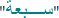137 661 87133 2917 Totals 131 14 270 3578

33-
The spelling of the word seven in verse 44 is not the same as the spelling of the same word in verse 87. Please see Table 4.
The word seven in verse 44 is spelt(sabaah). Its GV is 137
The word seven in verse 87 is spelt(sabaan). Its GV is 133
137 + 133 = 270
The 2 and the 7 (2 x 7) point to seven pairs (14).
So not only do the 2 sevens in the Sura add to 14, the sum of the GV values of their spelling also point to 14.

34- The number of verses between A.L.R (verse 1) and the first 7 (verse 44)
44 - 1 = 43
4 + 3 = 7
The number of verses between the first 7 (in verse 44) and the second 7 (in verse 87)
87 - 44 = 43
4 + 3 = 7
In other words, the first 7 (verse 44) lies exactly half way between A.L.R and the second 7 (verse 87). There are 43 verses on either side of the first 7.
The digits of the two number (4 and 3) and (4 and 3) = 14 (7 pairs)

35- The word God "Allah" appears only twice in Sura 15, in verses 69 and 96
(Table 5).
Between the first word "Allah" and the second "Allah" there are 27 verses.
96 - 69 = 27
2 x 7 = 14 (7 pairs)

-------------------Table 5
 Verse GV of the verse 69 1680 96 2407 Total 4087

38-
Between A.L.R (verse 1) and the first word "Allah" (verse 69) there are 68 verses.
69 - 1 = 68
6 + 8 = 14 (7 pairs)

39-
Between A.L.R. (verse 1) and the second word "Allah" (verse 96) there are 95 verses
96 - 1 = 95
9 + 5 = 14 (7 pairs)

40- Between the first 7 (verse 44) and the first "Allah" (verse 69) there 25 verses
69 - 44 = 25
2 + 5 = 7
Between the first 7 (verse 44) and the second "Allah" (verse 96) there are 52 verses
96 - 44 = 52
5 + 2 = 7
These two 7's give 14 (7 pairs)

41- The GV of verse 69 is 1680 (Table 5)
The GV of verse 96 is 2407
1680 + 2407 = 4087
4 + 0 + 8 + 7 = 19
If we add the number of verses in Sura 15 (99):
4087 + 99 = 4186
4 + 1 + 8 + 6 = 19

42- If we add the Sura number (15) to the GV of the two verses:
15 + 4087 = 4102
4 + 1 + 0 + 2 = 7
If we add the number of verses in the Sura:
4102 + 99 = 4201
4 + 2 + 0 + 1 = 7
Again these two 7's = 14 (7 pairs)

43- If we add the Sura number and the number of the two verses to their GV:
15 + 69 + 96 + 4087 = 4267
4 + 2 + 6 + 7 = 19
If we add to that the total number of verses in the Sura:
4267 + 99 = 4366
4 + 3 + 6 + 6 = 19

44- The next Table contains the 2 verses that contain the number 7 and also the 2 verses that contain the word God, as well as their GV's.

--------------------Table 6
 Verse GV 44 661 87 2917 69 1680 96 2407 Totals 296 7665

Verses total =
--296
GV total -----= 7665
-------------------7961 (19 x 419)

If we add the digits of the co-efficient (419):
4 + 1 + 9 = 14 (7 pairs).

45-
If we add to the previous total (7961) the Sura number (15) and the GV of the three words:(sabaah) and(sabaan) and Allah we get:
7961 + 15 + 137 + 133 + 66 = 8312
8 + 3 + 1 + 2 = 14 (7 pairs)

If we add the number of verses in the Sura (99) we get:
8312 + 99 = 8411
8 + 4 + 1 + 1 = 14 (7 pairs)

46- Verse 87 has 37 letters, and its GV is 2917 (Table 3)
If we place the Sura number, the verse number, the number of letters in the verse, and the GV of the verse side by side we get: (15) (87) (37) (2917)
1587372917 = 19 x 83545943

47- Sura 15 also contains the very significant verse:
Indeed, it is We who brought down the Reminder and We will surely preserve
it. 15:9
As we know, this verse which is God's promise to protect the Quran against any corruptions is strongly connected to the code 19.
The first half of verse 9 "it is We who brought down the Reminder" confirms that the Quran was revealed by God.
The second half of the verse "We will surely preserve it" provides the divine promise of protection.

The words
"These are the signs of the Book" appear after the abbreviated letters, at the beginning of 8 Suras. They are Sura numbers (10-12-13-15-26-27-28-31). Note that 19 is the 8th prime number.
Sura 15 is one of the 8 Suras.
In verse 1 we read "These are the signs of the Book"
In verse 9 we have the promise of preservation.
- The verse numbers 1 - 9 cannot escape our attention.
This confirms the strong link between the abbreviated letters, the number 19 and the protection of the Quran

48- The link between the abbreviated letters (verse 1) and the preservation of the Quran in verse 9 "We will surely preserve it" is also asserted by means of the number of letters as well as the GV:

----------------------------------Table 7
 Letters GV A.L.R 3 231 We will surely preserve it 11 1161 Total 14 1392

A.L.R.
= 3 letters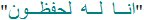(We will surely preserve it) = 11 letters.
3 + 11 = 14 (7 pairs)
Sura number (15) and Total GV (1392)
151392 = 19 x 7968

49-
The link is maintained if we incorporate the GV of the complete verse 9:

-----------Table 8
 GV A.L.R 231 Verse 9 2415 Total 2646

Sura number (15) followed by the total GV (2646)
152646 = 19 x 8034

50-
Let us take another look at Table 7
A.L.R. is 3 letters and with a GV of 231
"We will surely preserve it" has 11 letters and with a GV of 1161
3 + 231 + 11 + 1161 = 1406 (19 x 74)
The significance of the number 1406 and its coefficients (19 and 74) cannot be over emphasized:

1- 1406 is the number of years (Hijri) between the Revelation of the Quran (13 years before Hijra) and the discovery of the code 19 (1393 Hijri).
2- The number 19 appears only once in the Quran, and that is in Sura 74.
3- The code 19 was discovered in 1974.

51-
Now we can also add the number of letters in the phrase 7 of the pairs:
A.L.R. = 3 letters(We will surely preserve it) = 11 letters(7 of the pairs) = 13 letters
3 + 11 + 13 = 27
2 x 7 = 14 (7 pairs)

52-
Verse 9 contains 7 words, which is also the number of words in verse 87.
Seven words in each verse adds up to 14 (7 pairs)

--------------------------------------------

The code 19 and the role of the 7 pairs within the mathematical design of the Holy Quran were discovered by Rashad Khalifa, and thus we find the GV of Rashad Khalifa (1230) to be a component of the design in these glorious verses.

53-
------------------------Table 10
 GV A.L.R 231 We will surely preserve it 1161 Seven of the pairs 855 Rashad Khalifa 1230 Total 3477

3477 = 19 x 183

54-
As we have seen from point 33, and Table 4,
the word seven in verse 44 is spelt differently from the seven in verse 87
The sevenin verse 44 has a GV of 137
If we add the GV of the seven in verse 44 to the last total, we would get:
3477 + 137 = 3614
3 + 6 + 1 + 4 = 14 (7 pairs)

55- If we add the Sura number to the last total we get:
3614 + 15 = 3629
= 19 x 191

56- So far we looked at 6 verses in Sura 15, they are:
Verse 1 (A.L.R. the signs of the Quran)
Verse 9 (The preservation of the Quran)
Verses 44 and 87 (Where the number 7 is mentioned)
Verses 69 and 96 (Where the word God is mentioned)
The verse numbers, and their GV are in the following table:

--------------------Table 11
 Verse GV 1 2006 9 2415 44 661 87 2917 69 1680 96 2407 Total 306 12086

By adding the Sura number (15) to the above totals we get:
15 + 306 + 12086 = 12407
1 + 2 + 4 + 0 + 7 = 14 (7 pairs)
also 12407 = 19 x 653
6 + 5 + 3 = 14 (7 pairs)

57-
Next we add both, the Sura number (15) and the number of verses in the Sura (99):
15 + 99 + 306 + 12086 = 12506
1 + 2 + 5 + 0 + 6 = 14 (7 pairs)

58-
Next we add the GV of Rashad Khalifa (1230) to the totals in Table 11
306 + 12086 + 1230 = 13622
1 + 3 + 6 + 2 + 2 = 14 (7 Pairs)

59-
The GV of the 14 abbreviated letters is 693 (Table 1). These 14 letters are used in 14 different combinations. The GV of the 14 combinations of letters is 1709.
If we add the GV of Rashad Khalifa to the above numbers we get:
693 + 1709 + 1230 = 3632
3 + 6 + 3 + 2 = 14 (7 pairs)

The Quran and the number 14 (7 pairs)

1- The miracle of 19 was discovered in 1974, the first revelation of the Quran was in the year 610.
The time between the 2 dates is:
1974 - 610 = 1364
1 + 3 + 6 + 4 = 14 (7 pairs)

2- The words in 72:27 tell us that God does not reveal the ghayb (unseen) except to a messenger whom He chooses.
If we look at 72:27 we notice:
7 x 2 = 14 (7 pairs)
also 2 x 7 = 14 (7 pairs)
(14 letters + the 14 combinations)

3-
The role of the messenger who was destined to unveil the code of the Quran is stated in 3:81. Verse 3:81 is the 374th verse from the beginning of the Quran (7 + 286 + 81)
3 + 7 + 4 = 14 (7 pairs)

4-
The code of the Quran (19) appears only once in the Quran and that is in 74:30.
7 + 4 + 3 + 0 = 14 (7 pairs)

5-
The 14 letters are found at the beginning of 29 Suras. The GV of the 14 letters is 693.
29 + 693 = 722
7 x 2 x 2 = 14 x 2 (7 pairs x 2)
(14 letters + 14 combinations)

6-
GV of the 14 letters = 693
GV of the 14 combinations = 1709
GV of Rashad Khalifa = 1230
693 + 1709 + 1230 = 3632
3 + 6 + 3 + 2 = 14 (7 pairs)

7-
The first verse to contain abbreviated letters is 2:1, the last is 68:1. If we add these numbers we get:
2 + 1 + 68 + 1 = 72
7 x 2 = 14 (7 pairs)

8-
Among the combinations of letters (e.g. ALM, ALR, etc) there is only one combination that appears 7 times, and that is (Ha Mim). This combination has 2 letters. Each of the (7) Sura starts with (2) letters.
7 x 2 = 14 (7 pairs)

9-
These two letters (Ha) and (Mim) appear in the 7 Suras a total of 2147 times.
2 + 1 + 4 + 7 = 14 (7 pairs)

10-
The GV of the words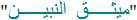(The Covenant of the prophets) which is mentioned in 3:81 is 793.
7 + 9 + 3 = 19
The Covenant of the prophets is the subject of 3:81 and 33:7
If we add these numbers to the GV of the messenger who is the subject of this Covenant (Rashad Khalifa), which is 1230, we get:
(3 + 81) + (33 + 7) + 793 + 1230 = 2147
= 19 x 113
Also, 2 + 1 + 4 + 7 = 14 (7 pairs)

11-
Finally, if we look at all the Suras whose digits add up to 14 (e.g. Sura 59, 5 + 9 = 14), then add the Sura and verse numbers we get the following:

-----------------Table 12
 Sura Verses in the Sura 59 24 68 52 77 50 86 17 95 8 385 151

Total of Sura numbers- = -385
Total number of verses- = 151
--------------------------------536
5 + 3 + 6 = 14 (7 pairs)

Conclusion
The facts above present indisputable evidence for the role of the 14 letters within the mathematical miracle of the Quran.
Having said that, it is important to note that it is the use of the 14 letters by God which presents the awesome designs and not the number 14 in itself.
14 (as a number) is not the code of the Quran, and should not be thought of in that light. The code of the Quran is 19 and no other.

Gematrical Values of the Arabic letters (Abjadiyah)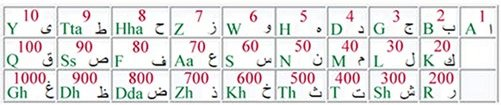Praise and Glory to God, the One, the Almighty